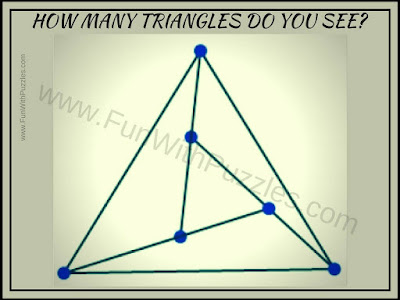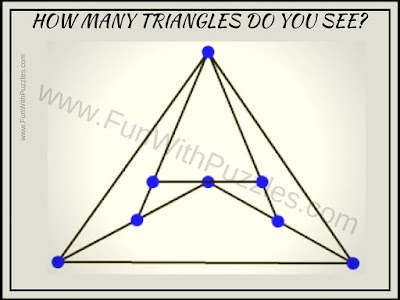Earlier we have posted finding the number of squares in the given picture which has got a very good response from the audience. So on a similar line, we are now posting the brain teasers in which one has to find the number of triangles in the given puzzle pictures.
There are series of puzzle images of triangles that are divided further into smaller triangles. One has to count all the big and small triangles in the given puzzle pictures. Getting the exact count will be the challenge here as one always tends to miss one of two triangles.
Answers to these puzzles will be provided at the end. Do post your answers in the comment section of this post.1. Can you count the number of Triangles in this Picture Puzzle?2. How many Triangles are there in this Picture?3. Do count the Number of Triangles in this Picture Image.4. How many total triangles can you see in this picture puzzle?5. Count total triangles in this Picture Puzzle.

Don't forget to check out similar Picture Puzzles and Brainteasers as mentioned below

List of Picture Puzzles and Brain Teasers

1. Picture Puzzles for Teens With Answers: Many easy to medium level picture puzzles that will test your observational skills.

2. Finding Hidden Faces in the Given Pictures Brain Teasers: Picture Puzzles in which your challenge to find hidden faces in the given picture puzzle images.

3. Interesting Brain Teasers to Challenge your Mind: This contains Picture Puzzles as well as Mathematical and Logical puzzles to train your brain.

4. Mind Twisting Out of Box Thinking Brain Teasers with Answers and Explanations: These are the Non-Verbal Puzzles that will make you think out of the box.

Answers the counting the number of triangles, brain teasers are as given below
1) 5

2) 6

3) 7

4) 7

5) 11